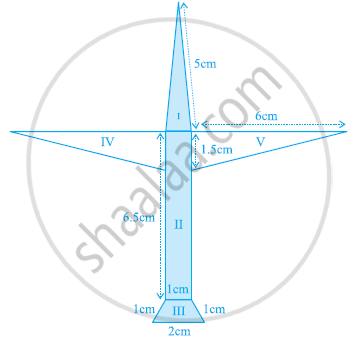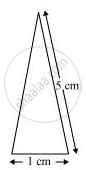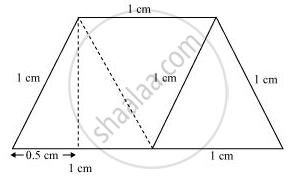# Radha Made a Picture of an Aeroplane with Coloured Papers as Shown in the Given Figure. Find the Total Area of the Paper Used. - Mathematics

Radha made a picture of an aeroplane with coloured papers as shown in the given figure. Find the total area of the paper used.#### SolutionFor triangle I

This triangle is an isosceles triangle.

Perimeter = 2s = (5 + 5 + 1) cm = 11cm

s = 11/2 = 5.5 cm

"Area of triangle "=sqrt(s(s-a)(s-b)(s-c))

=[sqrt(5.5(5.5-5)(5.5-5)(5.5-1))]cm^2

=[sqrt((5.5)(0.5)(0.5)(4.5))]cm^2

=0.75sqrt11cm^2

= (0.75 x 3.317) cm2

= 2.488 cm2  (Approximately)

Area = l × b = (6.5 × 1) cm= 6.5 cm2

"Perpendicular height of parallelogram "=(sqrt(1^2-(0.5)^2))cm = sqrt0.75 cm = 0.866 cm

Area = Area of parallelogram + Area of equilateral triangle

=(0.866)1+sqrt3/4(1)^2

= 0.866 + 0.433

= 1.299 cm2Area of triangle (IV) = Area of triangle in (V)

=(1/2xx1.5xx6)cm^2

= 4.5 cm2

Total area of the paper used = 2.488 + 6.5 + 1.299 + 4.5 × 2

= 19.287 cm2

Concept: Application of Heron’s Formula in Finding Areas of Quadrilaterals
Is there an error in this question or solution?

#### APPEARS IN

NCERT Class 9 Maths
Chapter 12 Heron's Formula
Exercise 12.2 | Q 3 | Page 206